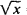# Equivalent Equations

## equivalent equations

[i¦kwiv·ə·lənt i′kwā·zhənz]
(mathematics)
Equations that have the same set of solutions.

## Equivalent Equations

equations that have the same solution sets; in the case of multiple roots, the multiplicities of the respective roots must be equal. Thus, of the three equations= 2, 3x – 7 = 5, and (x – 4)2 = 0, the first and second are equivalent but the first and third are not, since the multiplicity of the root x = 4, is equal to 1 for the first equation and 2 for the third equation.

If we add the same polynomial in x to both sides of a given equation or multiply both sides by a number other than zero, we obtain an equation equivalent to the given equation. For example, x2 - x + 1 = x – 1 and x2 - 2x + =0 are equivalent equations, since the polynomial - x + 1 has been added to both sides of the first equation. The equations 0.01 x2 – 0.37. x + 1 = 0 and x2 – 37x + 100 = 0 are also equivalent; here, both sides of the first equation have been multiplied by 100. If, however, we multiply or divide both sides of an equation by a polynomial of degree at least 1, then the resultant equation will in general not be equivalent to the original equation. For example, x – 1 = 0 and (x – 1) (x + 1) = 0 are not equivalent, since the root x = – 1 of the second equation is not a root of the first equation.

The concept of equivalent equations acquires a more precise meaning when the field in which the roots of the equations lie is indicated. For example, x2 - 1 = 0 and x4 – 1 = 0 are equivalent equations in the field of real numbers. The solution sets for both equations consist of two numbers: x, = 1 and x2 = – 1. The two equations, however, are not equivalent in the field of complex numbers, since the second equation now has two additional imaginary roots: x3 = i and x4 = – i.

The concept of equivalent equations can also be applied to a system of equations. For example, if P(x, y) and Q(x, y) are two polynomials in the variables x and y and if a, b, c, and d are real or complex numbers, then the two systems P(x, y) = 0, Q(x, y) = 0 and a P(x, y) + bQ(x, y) = 0, cP(x, y) + dQ(x, y) = 0 are equivalent when the value of the determinant adbc ≠ 0.

A. I. MARKUSHEVICH

References in periodicals archive ?
The equivalent equations of a single transmission line with dual transmission lines depicted by using admittance matrices  can be expressed as:
From Equations (1) and (2), we obtain two equivalent equations for [P.
For example, knowing that 3 + 2 = 5 and 3 = 5 - 2 are equivalent equations can help learners understand why x + 2 = 5 can be transformed to x = 5 - 2.
Her early geometric diagrams conjugated with their equivalent equations already evinced an attraction to the myriad virtual subsets lurking in the reputedly "simple and single" Minimalist object.
The above results indicate that the point estimates of the parameters in the monetary loss equivalent equations are not sensitive to the assumed degree of risk aversion, but the precision of the estimates are.

Site: Follow: Share:
Open / Close